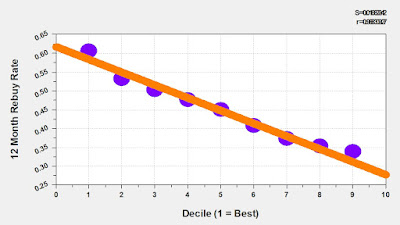## January 30, 2020

You'll end up with your equations for File Power (here's some SPSS code for your enjoyment).

compute predr = 0.
if (seg = 0) predr = -0.683 - 0.404*root_rec + 0.172*new + 0.219*exi + 0.161*abo + 0.122*bel
+ 0.052*kh01 + 0.168*ch01 - 0.371*ch03 - 0.574*mr00 + 0.105*mr01 + 0.066*mr02 + 0.265*mr03
+ 0.189*mr07 + 0.299*mr09.
if (seg = 1) predr = -0.152 - 0.356*root_rec + 0.113*new + 0.127*exi + 0.145*abo + 0.106*bel
+ 0.057*kh01 - 0.143*ch02 - 0.320*ch03 + 0.121*mr01 + 0.111*mr02 + 0.207*mr03 + 0.169*mr04
+ 0.099*mr06 + 0.232*mr07 + 0.274*mr09 + 0.162*mr10.
if (seg = 2) predr = -0.191 - 0.413*root_rec + 0.210*freq + 0.081*new + 0.218*exi + 0.070*bel
+ 0.090*kh01 - 0.119*ch02 - 0.267*ch03 + 0.069*mr01 + 0.058*mr02 + 0.109*mr03 + 0.166*mr04
+ 0.107*mr05 + 0.210*mr07 + 0.162*mr08 + 0.187*mr09 + 0.115*mr10.
if (seg = 3) predr = 1.869 - 0.772*root_rec + 0.165*bel + 0.058*ch01 - 0.208*ch03
- 0.091*mr00 + 0.061*mr02 + 0.138*mr03 + 0.135*mr07 + 0.072*mr09 - 0.132*ch02
+ 0.126*mr04 + 0.062*mr10 + 0.051*freq + 0.153*mr08.
compute predr = EXP(predr) / (1 + EXP(predr)).
compute preds = 0.
if (seg = 0) preds = 42.722 + 8.123*root_hst + 60.016*kh01 - 8.628*root_rec + 24.272*mr08
+ 6.848*ch02 - 12.104*mr02 + 5.336*bel - 9.537*mr01 + 0.109*history - 8.635*mr06 + 15.424*mr04.
if (seg = 1) preds = 26.817 + 5.158*root_hst + 57.236*kh01 - 10.018*root_rec + 26.155*mr08
+ 4.678*ch02 + 7.961*kh00 + 4.970*bel + 0.235*history + 21.957*mr05 + 11.116*mr07
+ 8.769*mr09 - 5.628*ch03 + 26.061*mr00.
if (seg = 2) preds = 21.571 + 4.219*root_hst + 50.386*kh01 - 9.942*root_rec + 19.582*mr08
+ 3.931*ch02 + 5.743*kh00 + 5.363*bel + 0.187*history + 9.192*mr04 + 17.595*mr05
+ 8.120*mr07 - 5.897*ch03 + 18.644*mr00 + 6.626*mr10 - 6.462*ch01.
if (seg = 3) preds = 27.397 + 3.665*root_hst + 52.893*kh01 - 19.142*root_rec + 14.774*mr08
+ 7.244*ch02 + 5.514*kh00 + 0.160*history - 6.150*mr06 + 8.442*mr05 + 7.073*mr07
+ 8.543*mr09 - 10.618*ch03 + 6.829*mr10.
if (preds le 30) preds = 30.
compute power = predr * preds.

Here's where the fun starts ... you can measure the incremental future value of various marketing activities and merchandising strategies via our File Power equations.

For instance, I can score File Power using the equations above. Then, for Merchandise Category = 03, I zero out the attribute ... essentially assuming that no customer ever purchased from the category. I re-run the customer file through the code, and sum File Power again.

The incremental difference between the two runs is the File Power attributable to Merchandise Category = 03.
• With Merchandise Category = 03:  File Power = 34,906,203. Average = 73.58.
• Without Merchandise Category = 03:  File Power = 34,560,078. Average = 72.85.
Notice that there is very little incremental File Power generated by this Merchandise Category. The category certainly generates short-term sales/profit (clearly important), but does not add to future File Power.

Ultimately, we're comparing what this Merchandise Category brings to the table vs. a "replacement category" ... it's comparable to baseball metrics where you compare the value of Christian Yelich vs. a replacement player from the AAA level. In that world, you see that Christian Yelich might be worth 7 wins a year. The team might win 96 games, and his incremental contribution is worth 7. A team full of replacement value players (i.e. AAA players) get you to 50-60 wins. Then your best players add incremental value ... if you have 25 players on a great team you get maybe 1.5 or 2.0 incremental wins per player.

That's what we're simulating with File Power. The equations play a key role in determining the incremental value of different aspects of the business.